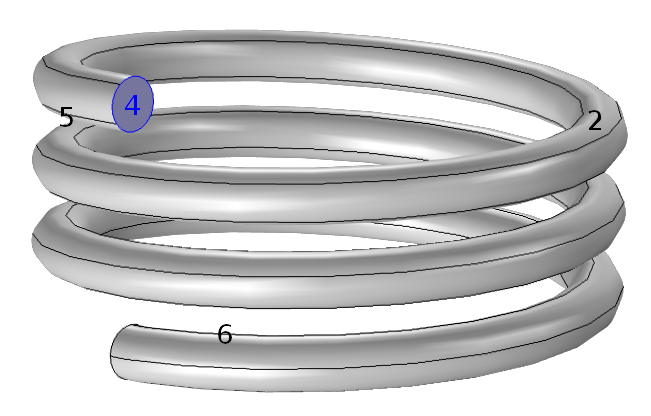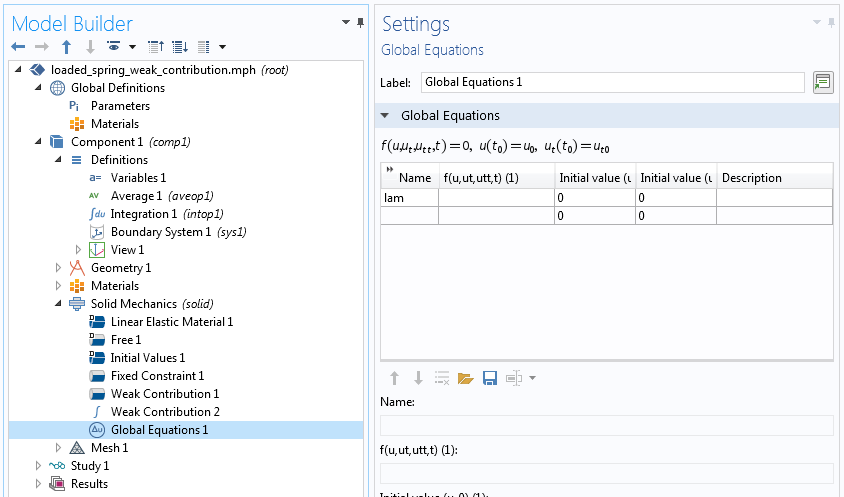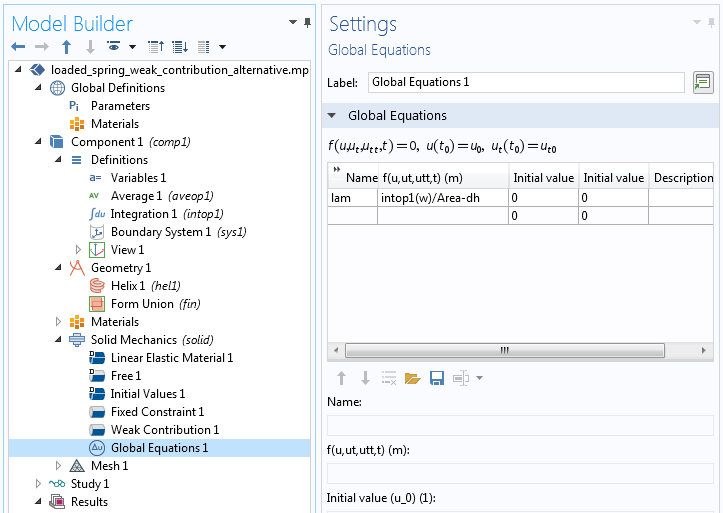# Nonstandard Constraints and the Power of Weak Contributions

October 16, 2018

Have you ever wanted to add a certain boundary or domain condition to a physics problem but couldn’t find a built-in feature? Today, we will show you how to implement nonstandard constraints using the so-called weak contributions. Weak contributions are, in fact, what the software internally uses to apply the built-in domain and boundary conditions. They provide a flexible and physics-independent way to extend the applicability of the COMSOL Multiphysics® software.

### Introduction to Weak Contributions

Many of the problems solved in COMSOL Multiphysics can be thought of as finding functions that minimize some quantity. In equilibrium problems of elasticity, for example, we look for displacements that minimize the total strain energy. In our blog series on variational problems and constraints, we showed how to use the Weak Form PDE interface to solve both constrained and unconstrained variational problems. We used a generalized constraint framework to deal with all kinds of restrictions on the solution. There, we showed you how to implement both the unconstrained and constrained problem.

Often, the quantity that has to be minimized is well understood and what we have to prescribe in our specific situations are the constraints. Ideally, we should not reinvent the wheel on the unconstrained problem. Frequently, constraints are boundary conditions, but sometimes they can be requirements to be satisfied at every point or by an integral of the solution. Several options for boundary conditions and other constraints are built into COMSOL Multiphysics, but from time to time, you may want to add a novel constraint or two. Today, we will see how to do so using weak contributions.

In this blog post, we:

• Give a quick recap of adding constraints to variational problems
• Use weak contributions to add a nonstandard constraint to a rather well-known equation
• Compare this strategy with a more physically motivated implementation

### Adding Constraints to an Extremization Problem

In our blog series on variational problems and constraints, we discussed in detail the analytical and numerical aspects of the subject as well as the COMSOL® software implementation. Readers unfamiliar with the subject will benefit from going over that series. In this section, we summarize the main ideas needed to work through today’s examples.

The method of Lagrange multipliers is used to recast constrained variational problems to equivalent unconstrained problems. Consider the constrained variational problem

(1)

\textrm{Find the function } u(x) \textrm{ that minimizes } E[u(x)] = \int_a^b F(x,u,u^{\prime})dx,

(2)

\textrm{subject to } g(x,u,u^{\prime})=0 \textrm{ for all } x.

The feasible critical points of this constrained problem are the stationary points of the augmented functional

(3)

E[u(x),\lambda(x)] = \int_a^b F(x,u,u^{\prime})dx+\int_a^b\lambda(x)g(x,u,u^{\prime})dx.

Let’s take the variational derivative of this functional.

(4)

\delta E = \delta \int_a^b F(x,u,u^{\prime})dx+\delta \int_a^b\lambda(x)g(x,u,u^{\prime})dx.

Say the unconstrained part of the problem is already taken care of and we just want to add what is necessary to enforce a constraint. Our responsibility then is only the second term in the above equation. In COMSOL Multiphysics, when we add a physics interface, it can be thought of as adding an unconstrained variational problem. Afterward, constraints on boundaries and domains can be added through one or several built-in standard boundary conditions. What if we have a nonstandard constraint that is not built in? Using weak contributions gives great flexibility to add such conditions.

Going back to the functional above, let us focus on the contributions coming from the constraint.

\delta E = \framebox{Something Built-in}+\delta \int_a^b\lambda(x)g(x,u,u^{\prime})dx = \framebox{Something Built-in}+ \int_a^b\left[\lambda \delta g+g\delta \lambda\right]dx .

For the distributed constraint above, the Lagrange multiplier \lambda (x) is a function defined over the geometric entity subject to the constraint. For a global constraint such as an integral or average constraint, on the other hand, the Lagrange multiplier is one number. Say we want to impose the global integral constraint

\int_a^bg(x,u,u^{\prime})dx-G = 0.

The augmented functional is

E=\framebox{Something Built-in} + \lambda\left[\int_a^bg(x,u,u’)dx – G\right]

and its variation is

\delta E=\framebox{Something Built-in} + \lambda\int_a^b\delta g(x,u,u^{\prime})dx + \delta \lambda\left[\int_a^bg(x,u,u’)dx – G\right] ,

(5)

\delta E=\framebox{Something Built-in} + \lambda\int_a^b\left[\frac{\partial g}{\partial u}\delta u + \frac{\partial g}{\partial u’}\delta u’\right]dx + \delta \lambda\left[\int_a^bg(x,u,u’)dx – G\right].

Thus, if the boundary condition or other constraint you want to enforce on your solution is not built in, but there is a built-in physics interface for the physics, all you need to do is add the last two terms in the above equation using the Weak Contribution node. Let’s demonstrate this with an example.

### Constraining the Average Vertical Displacement of a Spring

In this example, a spring is rigidly fixed at the bottom end and we want the top end (boundary 4 in the model below) to have an average vertical displacement of 2 cm. This is a linear elasticity problem and this physics is built in. Also, rigidly fixing a face is a standard boundary condition. On the other hand, specifying an average displacement on a face is not.Note that we are not asking for the vertical displacement of each point on the face to be 2 cm. That could have been specified with the built-in Prescribed Displacement node. What we have is the global constraint

(6)

\frac{\int_A wdA}{\int_A dA}-dh = 0,

where A is the face in question and dh is the desired average vertical displacement (2 cm in our case). Here, dh is not a differential of any quantity. It is just the name of a parameter used for the average vertical displacement on the face. We could directly have written 2 cm in its place.

With all but this constraint implemented using standard features, our variational problem becomes finding the stationary point of the augmented functional

\framebox{Something Built-in} + \lambda\left[\frac{1}{A}\int_A wdA – dh\right].

The corresponding stationary condition is

(7)

\framebox{Something Built-in} + \lambda\left[\frac{1}{A}\int_A \delta wdA\right] + \delta \lambda\left[\frac{1}{A}\int_A wdA – dh\right] = 0, \quad \forall \quad \delta u, \delta v, \delta w, \delta \lambda.

Let us add these two contributions using a boundary weak contribution and a global weak contribution. In the Model Builder, we can distinguish between boundary and global weak contribution from the icons. Boundary contributions have the same icons as boundary conditions whereas global contributions have icons with an integral sign (\int). Additionally, the Settings window for boundary contributions contains a boundary selection section whereas there is no geometric entity selection for a global contribution.

Boundary and global weak contributions to enforce constraint on an average displacement over a surface.

Finally, the variable \lambda is an auxiliary global variable we defined in our Lagrange multiplier method. Any new variable related to the constraint has to be defined either in the Auxiliary Variable subnode of a Weak Contribution node or in the Global Equations node based on the nature of the constraint.

In our example, we have a global constraint and, as such, we have to define it using a Global Equations node. Often, an equation will be entered in the Global Equations Settings window as well. This is not necessary here, as we have included in the weak contribution a term containing the variation of the Lagrange multiplier. An alternative — using the Global Equation node to define both the global degree of freedom and its equation — will be discussed later. A global equation adds one degree of freedom to our problem.Defining an auxiliary global unknown.

If we solve this problem, we get the solution shown below. We can see the value of the Lagrange multiplier and the average displacements in Results > Derived Values. If we look at the vertical displacement on the constrained surface, it is not uniform; it just averages to 2 cm as per the constraint.

We would like to clarify two items about the above implementation.

1. Both the second and third terms in (Eq. 7) contain integrals. In the boundary weak contribution, Weak Contribution 1, we add just the integrand. The integral in the global weak contribution, on the other hand, needs the integration operator to be explicitly called. Alternatively, we could have added the integrand test(lam)*w/Area to Weak Contribution 1 and kept only -test(lam)*dh in Weak Contribution 2.
2. The constraint in this example is global. For a distributed constraint, the Lagrange multiplier is a function of location and it has to be defined as an auxiliary variable under the boundary weak contribution. See our blog post on variational constraints for more on this distinction.

#### Alternative Implementation

The term multiplying the variation of the Lagrange multiplier, \delta \lambda, can be specified in the Global Equation node, where the Lagrange multiplier itself is defined. The screenshots below show how to do so. This only replaces the third term in (Eq. 7). The term not containing \delta \lambda still has to be specified as a boundary weak contribution. Note that intop1() is an integration operator defined over boundary 4 and Area is the area of that boundary given by  intop1(1).Alternative specification of the weak term containing a variation of a global Lagrange multiplier.

### Physical Interpretation of the Lagrange Multiplier

The above solution gives us the displacements and stresses induced by moving boundary 4 by an average vertical displacement of 2 cm. The question is: How do we physically force the structure to conform to our wish? You guessed it: We apply a force. The Lagrange multiplier is related to the force (flux) needed to enforce a constraint. The operative word here is related. Let us see what we mean here in detail. First, let’s try an alternative formulation of the constraint.

The constraint in (Eq. 6) is mathematically equivalent to

(8)

The augmented functional corresponding to this form of the constraint is

\framebox{Something Built-in} + \lambda\left[\int_A wdA – Adh\right],

and the corresponding stationary condition is

(9)

\framebox{Something Built-in} + \lambda\left[\int_A \delta wdA\right] + \delta \lambda\left[\int_A wdA – Adh\right] = 0, \quad \forall \quad \delta u, \delta v, \delta w, \delta \lambda.

If we enter the last two terms in this equation as weak contributions and solve, we get a Lagrange multiplier much different from what was obtained in our first implementation. The displacements and stresses remain the same nevertheless. So, we can suspect that the Lagrange multiplier in and of itself is not a physical quantity and, as such, cannot tell us what to physically do to enforce a desired constraint. One reliable way to find out what we should do physically is to postprocess the results to see reaction forces (fluxes).

To rigorously establish what the Lagrange multiplier is physically, we have to look at the unconstrained part of the equation that we have been hiding so far. In today’s example, that means looking at the weak form of the solid mechanics equation. For a deformable solid in equilibrium, the weak form, also known as the virtual work equation, is given by

(10)

-\int_V\sigma:\delta \varepsilon dV +\int_V\mathbf{b}\cdot\delta\mathbf{u} dV+\int_A\mathbf{\Gamma}\cdot\delta\mathbf{u}dA =0 \quad \forall \delta\mathbf{u},

where \mathbf{u} = (u,v,w) is the displacement vector and \sigma, \varepsilon, \mathbf{b}, and \mathbf{\Gamma} are respectively the stress, strain, body load per unit volume, and boundary load. The weak form for any COMSOL Multiphysics physics interface can be viewed by enabling the Equation View.

If we compare (Eq. 10) with (Eq. 9) and (Eq. 7), we see that the Lagrange multipliers in today’s example appear in the same place as the boundary load on the constrained surface. One difference is that the Lagrange multiplier appears outside the surface integral, whereas in the boundary load is inside the integral. This stems from the global nature of our constraint. A second difference is the Lagrange multiplier in our example goes with the vertical displacement w, whereas the surface load in the solid mechanics equation is dot multiplied by the variation of the displacement vector. Let us reconcile these items one at a time.

\int_A\mathbf{\Gamma}\cdot\delta\mathbf{u}dA = \int_A\left[\Gamma_x\delta u + \Gamma_y\delta v + \Gamma_z\delta w\right]dA.

Now we see that our Lagrange multiplier is related to the vertical component of a boundary load. Finally, if the boundary load is constant, we have

\int_A\Gamma_z\delta wdA = \Gamma_z\int_A\delta wdA .

Comparing this with (Eq. 7), we see that in our first implementation, the Lagrange multiplier corresponds to the total vertical boundary load. Now that we know for the specific physics and a specific form of the constraint equation, the Lagrange multiplier is the total vertical load on a face, we can use the built-in Boundary Load node to enforce the constraint instead of the weak contribution. This process is shown in the loaded spring example in the Application Gallery.

Alternative implementation when we know what the Lagrange multiplier physically corresponds to.

This last implementation can be thought of as asking the software to apply whatever vertical total force is required to enforce an average vertical displacement. We could do that because, by looking at the weak form of the solid mechanics equation, we identify the correspondence between the Lagrange multiplier and the total force. It is not always possible to make such connections with a standard force (flux) term. Note that we could have used the default distributed boundary load, which would only have changed the number of the extra degrees of freedom used internally.

Generically speaking, for dimensional consistency, in (Eq. 3), the product of the Lagrange multiplier and the constraint g should give the density of the “energy” E per unit volume, area, or length depending on the geometric entity the constraint is applied on. In many engineering problems, E literally represents energy and, as such, we say the Lagrange multiplier is energetically conjugate with the constraint. This means, for example, that if we scale, square, or do any operation that mathematically changes the unit of the constraint equation, then the unit and thus the physical meaning of the Lagrange multiplier changes. What doesn’t change, however, is that of \lambda \frac{\partial g}{\partial u}, as it is always energetically conjugate with u (Eq. 5). It is this product of the Lagrange multiplier and the constraint Jacobian that is a force (flux) density in a generalized sense. If \frac{\partial g}{\partial u}=1, which is often the case with linear constraints, the Lagrange multiplier is indeed the generalized force (flux).

The beauty of the weak contribution is that you can enforce the constraint without having to go through the weak form of the built-in physics. Then, you can postprocess the result to find out the physical course of action. The implementation is physics independent.

### Concluding Thoughts on Weak Contributions

Today, we have discussed how the COMSOL Multiphysics software facilitates the implementation of nonstandard boundary conditions. Using weak contributions, we have a flexible and physics-independent strategy to add constraints that are not used frequently enough to be standard features in the software. The mathematical roots of this method are in problems where the solution minimizes some quantity, but the strategy can be used for problems given by partial differential equations that do not have a corresponding variational solution. For more background information on this topic, we recommend our blog series on the weak form and on variational problems.

### Next Steps

If you have any questions on weak contributions or another topic, or want to learn more about how the features and functionality in COMSOL Multiphysics suit your modeling needs, you’re welcome to contact us.

Check out the following Application Gallery examples and blog posts for more demonstrations of using weak contributions and extra equations in various physics areas: